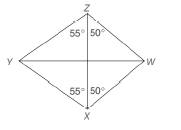Chapter 4.2, Problem 8EElementary Geometry For College St...

7th Edition
Alexander + 2 others
ISBN: 9781337614085

Solutions

Chapter
SectionElementary Geometry For College St...

7th Edition
Alexander + 2 others
ISBN: 9781337614085
Textbook Problem

In quadrilateral W X Y Z , the measures of selected angles are shown.a) What type of quadrilateral is W X Y Z ?b) Which diagonal of the quadrilateral has the greater length?To determine

The type of the quadrilateral WXYZ.

Explanation

Given,

From the above given figure

We have

mYZX=mYZX=55°,

mWZX=mWXZ=50°.

Thus,

mYZW=mYZX+mWZX

=55°+50°

To determine

b)

The diagonal of the quadrilateral that has greater length.

Still sussing out bartleby?

Check out a sample textbook solution.

See a sample solution

The Solution to Your Study Problems

Bartleby provides explanations to thousands of textbook problems written by our experts, many with advanced degrees!

Get Started

Explain why the formula for sample variance is different from the formula for population variance.

Essentials of Statistics for The Behavioral Sciences (MindTap Course List)

(2, 5]

Applied Calculus for the Managerial, Life, and Social Sciences: A Brief Approach

Evaluate the expression sin Exercises 116. (2)3

Finite Mathematics and Applied Calculus (MindTap Course List)

An integral for the solid obtained by rotating the region at the right about the y-axis is:

Study Guide for Stewart's Single Variable Calculus: Early Transcendentals, 8th

, where E is the wedge-shaped solid shown at the right, equals:

Study Guide for Stewart's Multivariable Calculus, 8th